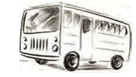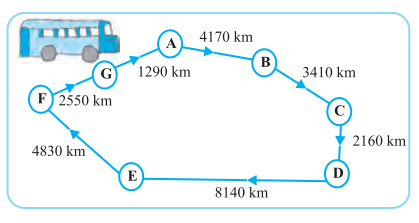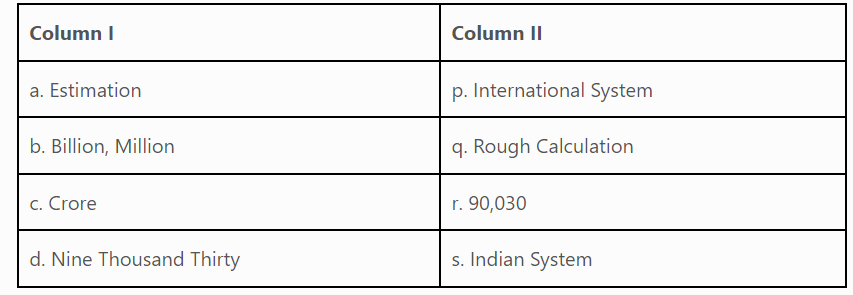Daily Practice Problems
Class 6 Maths
Knowing Our NumbersQuestion 1:

Can you instantly find the greatest and the smallest numbers in each row?

1. 382, 4972, 18, 59785, 750.

2. 1473, 89423, 100, 5000, 310.

3. 1834, 75284, 111, 2333, 450.

4. 2853, 7691, 9999, 12002, 124.

Question 2:

A bus started its journey and reached different places with a speed of 60 km/hour. The journey is shown fig.(i) Find the total distance covered by the bus from A to D.

(ii) Find the total distance covered by the bus from D to G.

(iii) Find the total distance covered by the bus, if it starts from A and returns back to A.

(iv) Can you find the difference of distances from C to D and D to E?Question 3:

In one state, the number of bicycles sold in the year 2002-2003 was 7,43,000. In the year 2003-2004, the number of bicycles sold was 8,00,100. In which year were more bicycles sold and how many more?

Question 4:

Estimate: 5,290 + 17,986.

Question 5:

Write the expressions for each of the following using brackets.

(a) Four multiplied by the sum of nine and two.

(b) Divide the difference of eighteen and six by four.

(c) Forty-five divided by three times the sum of three and two.

Question 6:

Fill in the blanks:

(a) 1 million = _____ hundred thousand.

(b) 1 crore = _____ ten lakhs.

(c) 1 crore = _____ million.

(d) 1 million = _____ lakh.

Question 7:

Match the following:Question 8:

Write the smallest and the greatest 5-digit numbers using the digits 0,2,4,6,8 (Repetition of digits is not allowed).

Question 9:

Kirti Bookstore sold books worth Rs.2,85,891 in the first week of June and books worth Rs.4,00,768 in the second week of the month. How much was the sale for the two weeks together? In which week was the sale greater and by how much?

Question 10:

Write in Roman Numerals

(a) 69

(b) 98.

Question 11:

Round off the given numbers to the nearest tens, hundreds and thousands.

(a) 75847, round off to nearest tens ________________

(b)75847, round off to nearest hundreds ________________

(c) 75847 round off to nearest thousands _________

Question 12:

Write and solve the expression: Thirteen multiplied by sum of four and eleven. Now reverse the result and add it to earlier result, what you obtain multiply it by 13.

Question 13:

What is the successor of greatest 7-digit number?

Question 14:

(i) 527864 (ii) 95432 (iii) 18950049 (iv) 70002509

Arrange these in ascending and descending order.

Question 15:

How many crores makes a billion?

Question 16:

What is the place value of 7 in 2734?

Question 17:

There are two factories located at place P and the other at place Q. From these factories, a certain commodity is to be delivered to each of the depots situated at A, B and C. Weekly production of commodity by P and Q are 120 kg and 150 kg respectively. Weekly requirement of commodity by A, B and C are 80 kg, 90 kg and 100 kg respectively. P delivers 60 kg to A, 40 kg to B and 20 kg to C. How much amount of the commodity should Q deliver to A, B and C to meet their requirement? If the rate of the commodity is Rs 20 per kg, find the total amount to be paid to P and Q.

Question 18:

The town newspaper is published every day. One copy has 12 pages. Everyday 11,980 copies are printed. How many total pages are printed every day?

Question 19:

Use the given digits without repetition and make the greatest and smallest 4-digit numbers.

(a) 2, 8, 7, 4

(b) 9, 7, 4, 1

Question 20:

Write True/False for the following statements:

(a) Roman symbol X cannot be repeated more than three times

(b) VXXX = 25.

(c) Estimate value of274 rounding off to nearest hundreds = 200.

(d) I and X can repeat at the most three times.

(e) V, L and D are neither, repeated nor written to the left of greater value symbol.

(f) There are six basic symbols in Roman Numeration system.

************